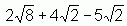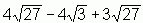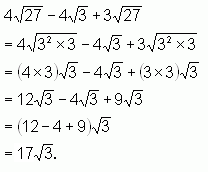SEARCH HOMEMath Central Quandaries & QueriesQuestion from Ciara, a student: How would you calculate 2 to the square root of 8 plus 4 to the square root of 2 minus 5 to the square root of 2?Hi Ciara.

I think you are asking how to simplify this:(if I'm wrong, then let me know).

I'll show you how this is done using a similar question:The important thing to remember is that just as you cannot combine 2x + 3y into 5xy, you cannot combine radical expressions unless the figure under the radical signs are the same. So you need to see if you can simplify what is under the radical (we call the figure under the radical sign the radicand).

To do this, you should look for factors that are perfect squares. I know that 27 = 9 x 3 and 9 is a perfect square because 9 = 32.

Then you can remove the factors that are squares by taking them outside the radical as factors.

I'll show you how this is solved completely:You see, once you have the same radicands (the fourth line is just a series of terms all with 3 under the radical sign), you can combine them. Think of how you do it with variables: 12y - 4y + 9y = 17y.

You can solve your question the same way, Ciara.

Cheers,
Stephen La Rocque.Math Central is supported by the University of Regina and The Pacific Institute for the Mathematical Sciences.